Type
Quiz
Book Title
Heat and Mass Transfer: Fundamentals and Applications 6th Edition
ISBN 13
978-0073398198

### 978-0073398198 Chapter 4 Part 3

August 3, 2020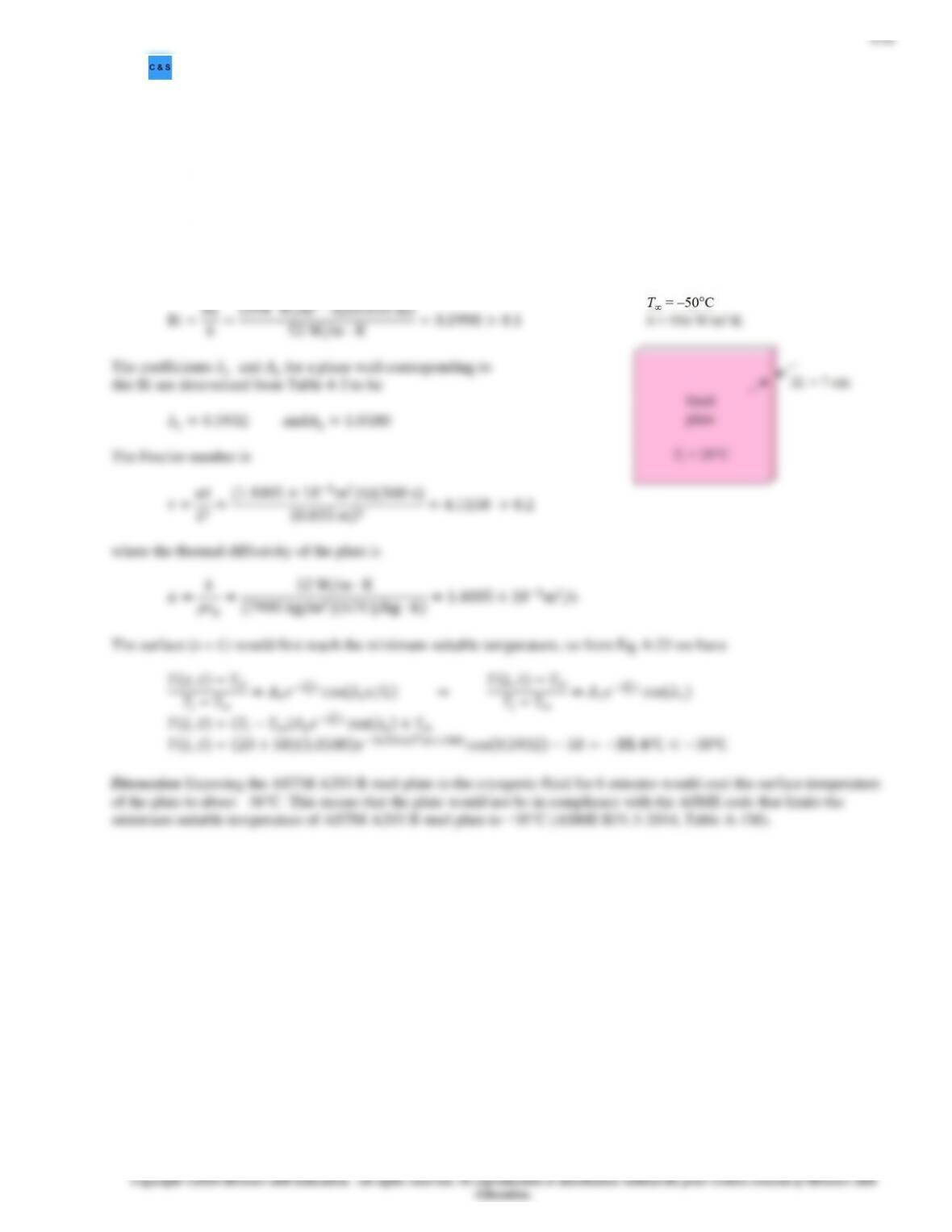4-57 ASTM A203 B steel plate is exposed to cryogenic fluid at T = 50°C and h = 594 W/m2·K. The plate has an
initial temperature of 20°C. Would the plate comply with the ASME Code for Process Piping if it is exposed to the cold fluid
for 6 minutes?
Assumptions1 Heat conduction is transient and one dimensional. 2 Thermal properties are constant. 3 Radiation effects are
negligible. 4 The convection heat transfer coefficient is constant. 5 The Fourier number is > 0.2 so that the one term-term
approximate solutions are applicable.
Properties The thermal properties given are cp = 470 J/kg·K, k = 52 W/m·K, and ρ = 7900 kg/m3.
Analysis The plate is considered as a large plane wall with a thickness of 2L = 7 cm subjected to convection on both sides.
The Biot number for this process is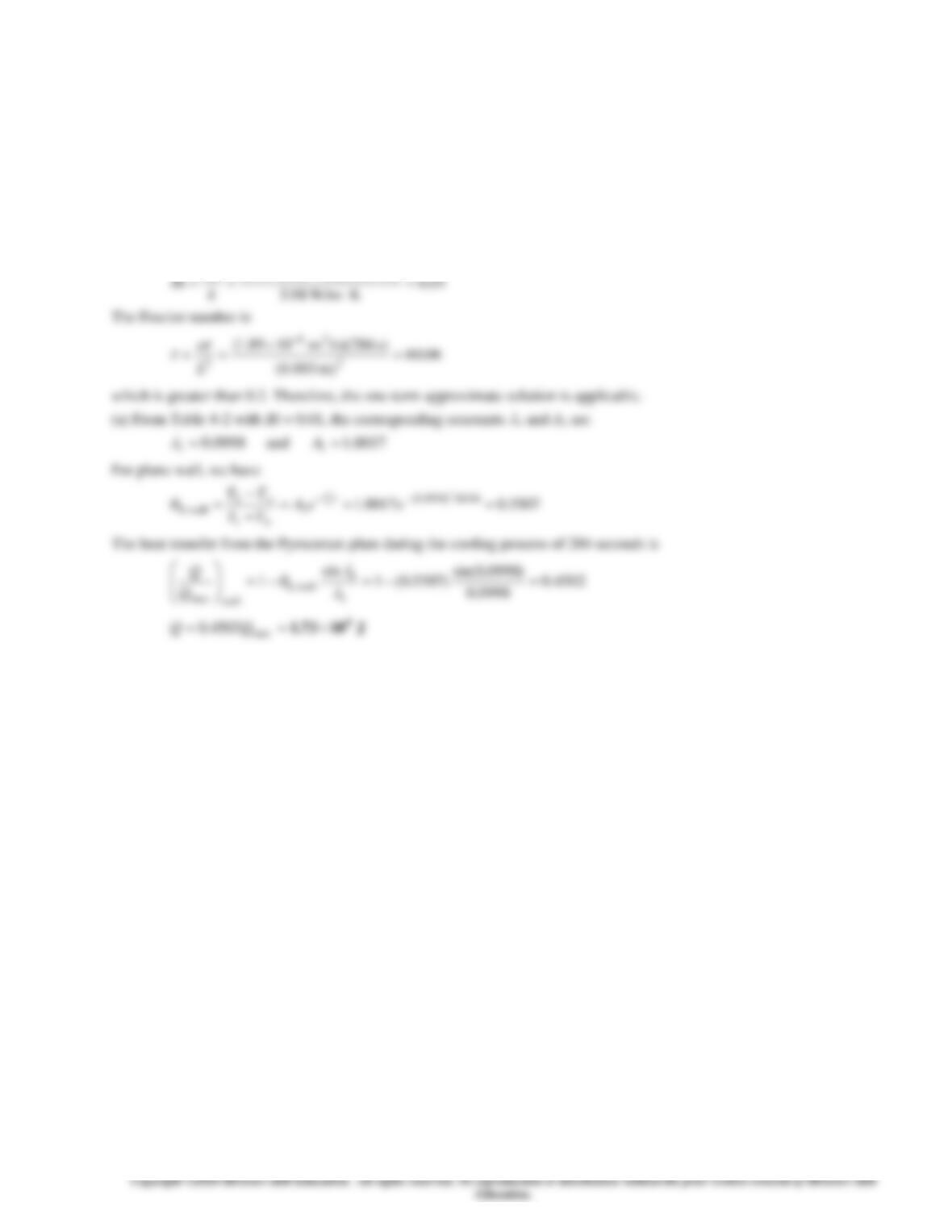4-42
4-58 The heat transfer from the Pyroceram plate during the cooling process of 286 seconds is to be determined using the one-
term approximate solutions.
Assumptions 1 Heat conduction is one-dimensional. 2 Thermal properties are constant. 3 Convection heat transfer coefficient
is uniform. 4 Heat transfer by radiation is negligible.
Properties The properties of the Pyroceram plate are given as
= 2600 kg/m3, cp = 808 J/kg ∙ K, k = 3.98 W/m ∙ K, and
=
1.89 10−6 m2/s.
Analysis The maximum amount heat transfer from the Pyroceram plate is
J 10838.3K )25500)(KJ/kg 808)(kg 10()( 6
max ===
TTmcQip
The Biot number for this process is
)m 003.0)(K W/m3.13(2
hL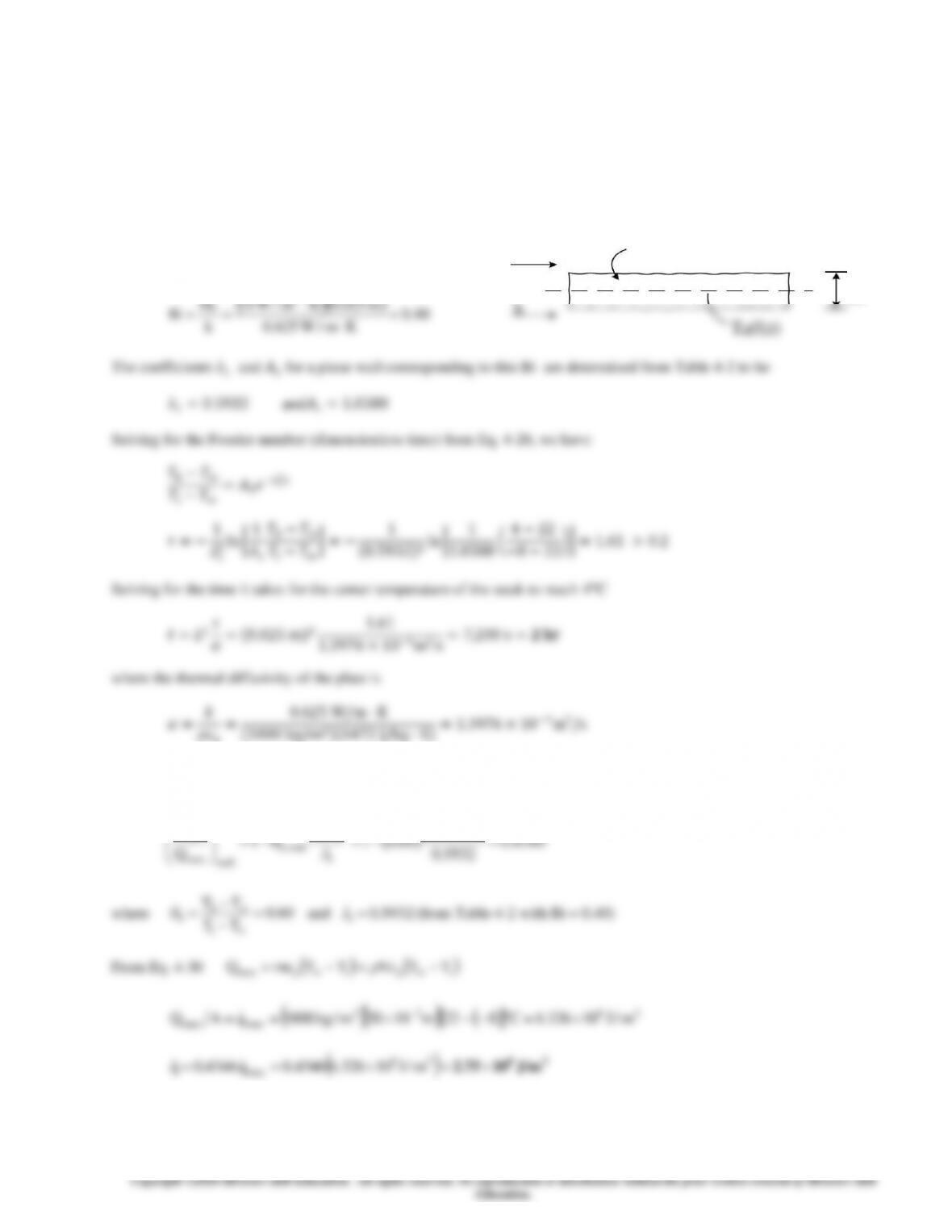4-43
4-59 The time required and the amount of energy removed to thaw a frozen steak to a specified temperature is to be
determined.
Assumptions 1 Heat conduction is transient and one dimensional. 2 Thermal properties are constant. 3 Radiation effects are
negligible. 4 The convection heat transfer coefficient is constant. 5 Neglect the heat of fusion associated with the melting
phase change 6 The Fourier number is > 0.2 so that the one term-term approximate solutions are applicable.
Properties The thermal properties given are cp = 4472 J/kg·K, k = 0.625 W/m·K, and ρ = 1000 kg/m3.
Analysis (a) The steak is considered as a large plane wall
with a thickness of 2L = 50mm subjected to convection on
both sides. The Biot number for this process is
(b) To calculate the amount of energy per unit area that has been removed from the steak during this period of thawing, use
Eq. 4-33,
5932.0sin
sin
Q
1
(c) Since Bi > 0.1, lumped system analysis is not appropriate or internal thermal resistance cannot be neglected.
2
T
Ti
2L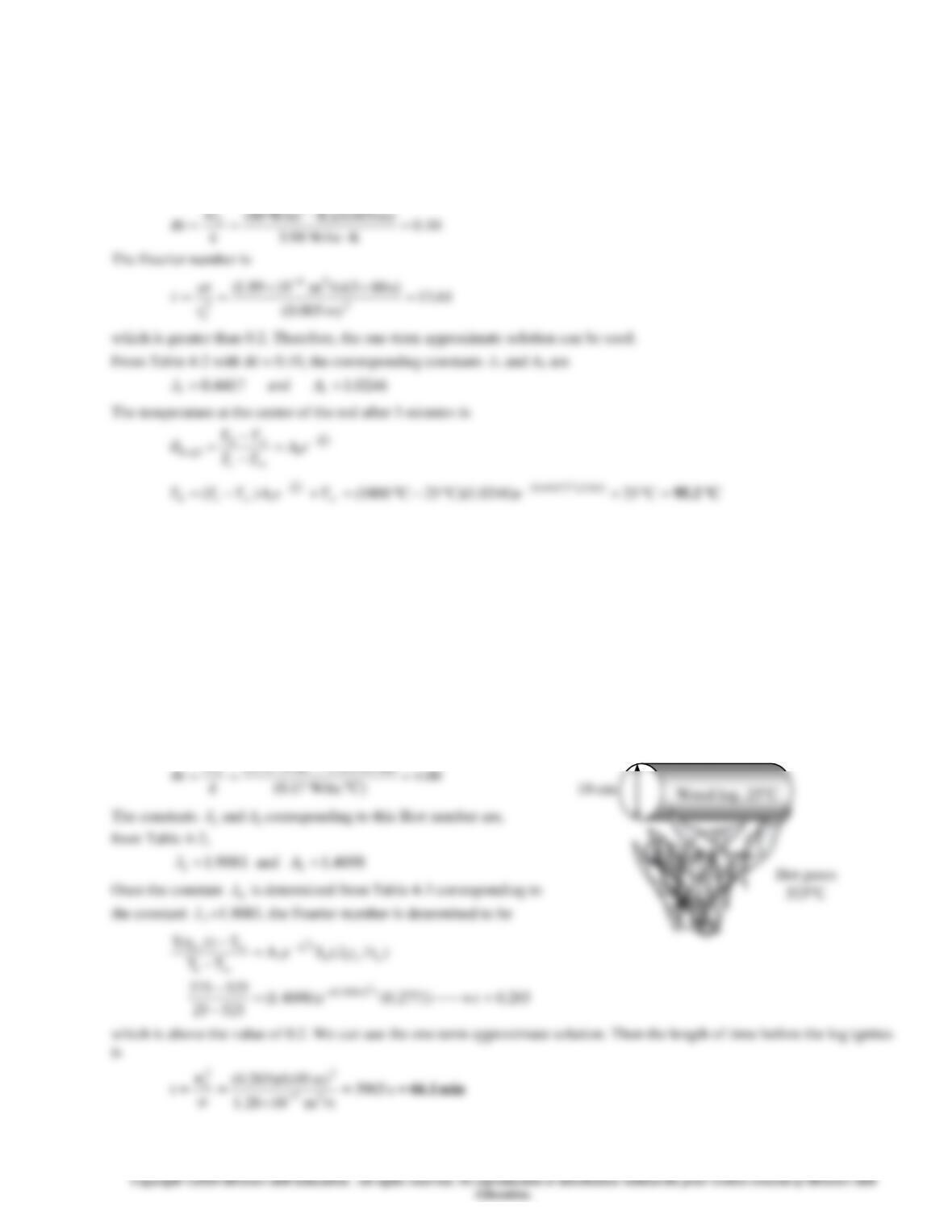4-44
4-60 The temperature at the center of a Pyroceram rod after 3 minutes of cooling is to be determined using analytical one-
term approximation method.
Assumptions 1 Heat conduction is one-dimensional. 2 Thermal properties are constant. 3 Convection heat transfer coefficient
is uniform. 4 Heat transfer by radiation is negligible.
Properties The properties of Pyroceram rod are given as
= 2600 kg/m3, cp = 808 J/kg ∙ K, k = 3.98 W/m ∙ K, and
= 1.89
10−6 m2/s
Analysis The Biot number for this process is
4-61 A long cylindrical wood log is exposed to hot gases in a fireplace. The time for the ignition of the wood is to be
determined.
Assumptions 1 Heat conduction in the wood is one-dimensional since it is long and it has thermal symmetry about the center
line. 2 The thermal properties of the wood are constant. 3 The heat transfer coefficient is constant and uniform over the entire
surface. 4 The Fourier number is > 0.2 so that the one-term approximate solutions are applicable (this assumption will be
verified).
Properties The properties of wood are given to be k = 0.17 W/m.C, = 1.2810-7 m2/s
Analysis The Biot number is
)m 05.0)(C. W/m6.13(2
hr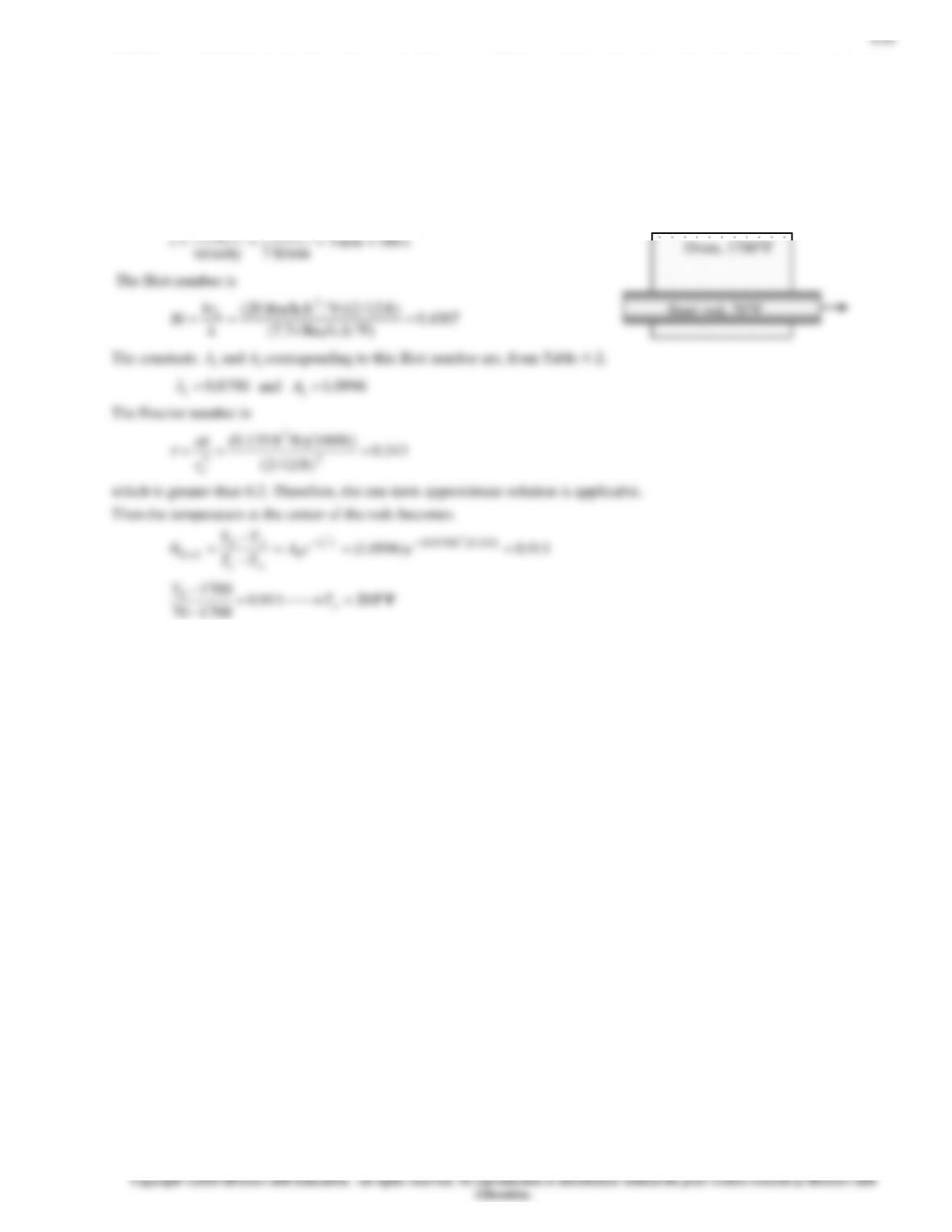4-62E Long cylindrical steel rods are heat-treated in an oven. Their centerline temperature when they leave the oven is to be
determined.
Assumptions 1 Heat conduction in the rods is one-dimensional since the rods are long and they have thermal symmetry about
the center line. 2 The thermal properties of the rod are constant. 3 The heat transfer coefficient is constant and uniform over
the entire surface. 4 The Fourier number is > 0.2 so that the one-term approximate solutions are applicable (this assumption
will be verified).
Properties The properties of AISI stainless steel rods are given to be k = 7.74 Btu/h.ft.F, = 0.135 ft2/h.
Analysis The time the steel rods stays in the oven can be determined from
ft 21
length ===t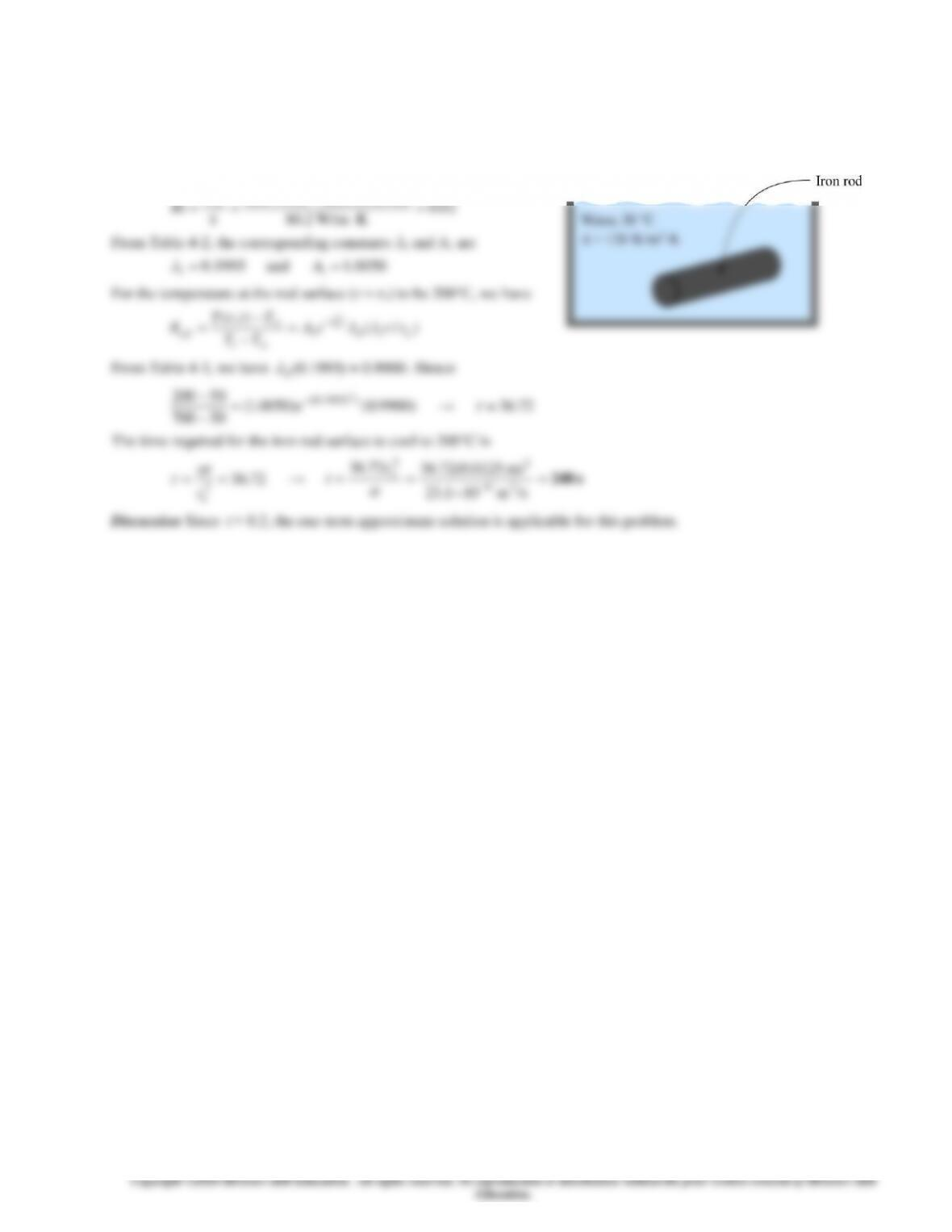4-46
4-63 The time required for a long iron rod surface temperature to cool to 200°C in a water bath is to be determined.
Assumptions 1 Heat conduction is one-dimensional. 2 Thermal properties are constant. 3 Convection heat transfer coefficient
is uniform. 4 Heat transfer by radiation is negligible.
Properties The properties of iron rod are given as
= 7870 kg/m3, cp = 447 J/kg ∙ K, k = 80.2 W/m ∙ K, and
= 23.1 10−6
m2/s.
Analysis The Biot number for this process is
)m 0125.0)(K W/m128(2
hr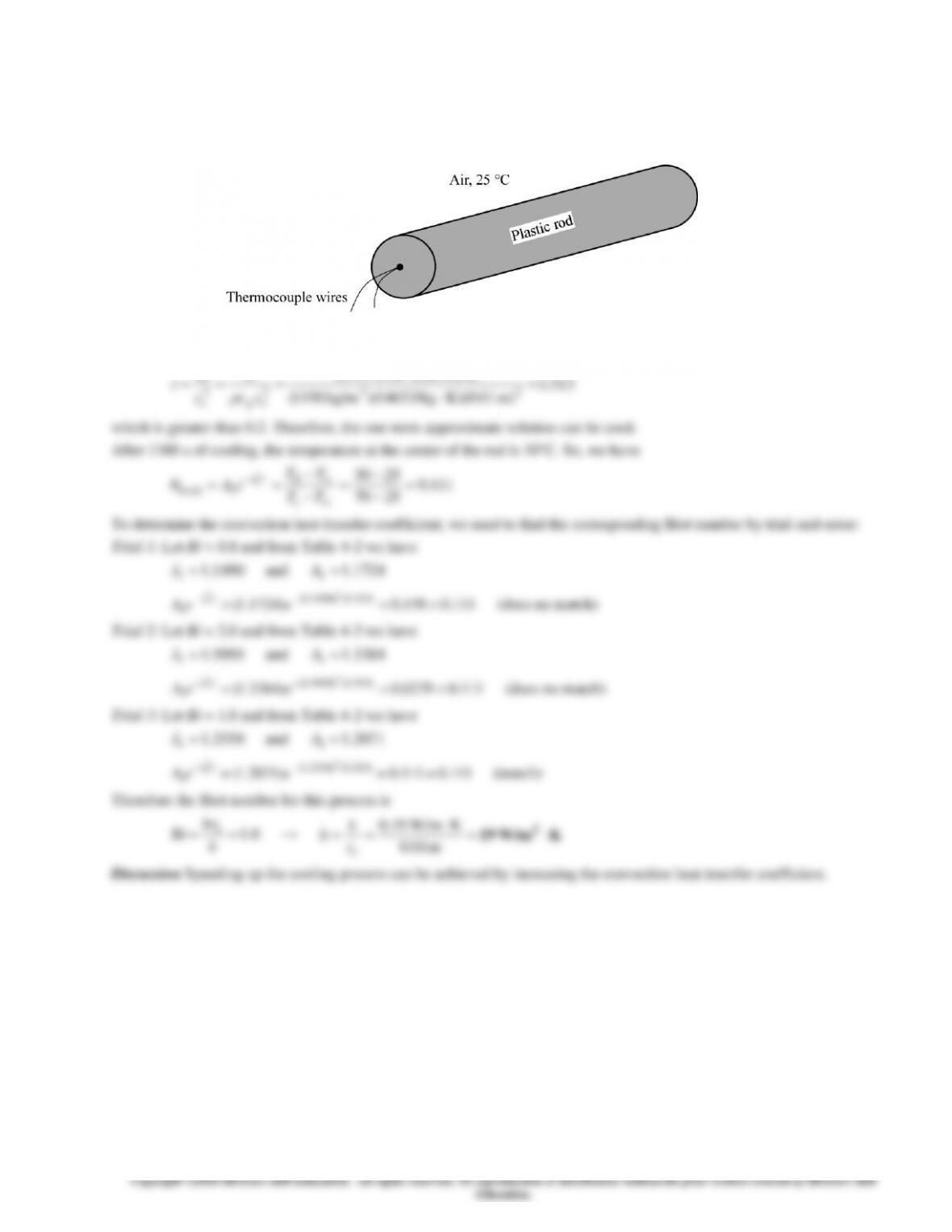4-47
4-64 The convection heat transfer coefficient for a plastic rod being cooled is to be determined.
Assumptions 1 Heat conduction is one-dimensional. 2 Thermal properties are constant. 3 Convection heat transfer coefficient
is uniform. 4 Heat transfer by radiation is negligible.
Properties The properties of the plastic rod are given as
= 1190 kg/m3, cp = 1465 J/kg ∙ K, and k = 0.19 W/m ∙ K.
Analysis The Fourier number is
)s 1388)(K W/m19.0(
kt
t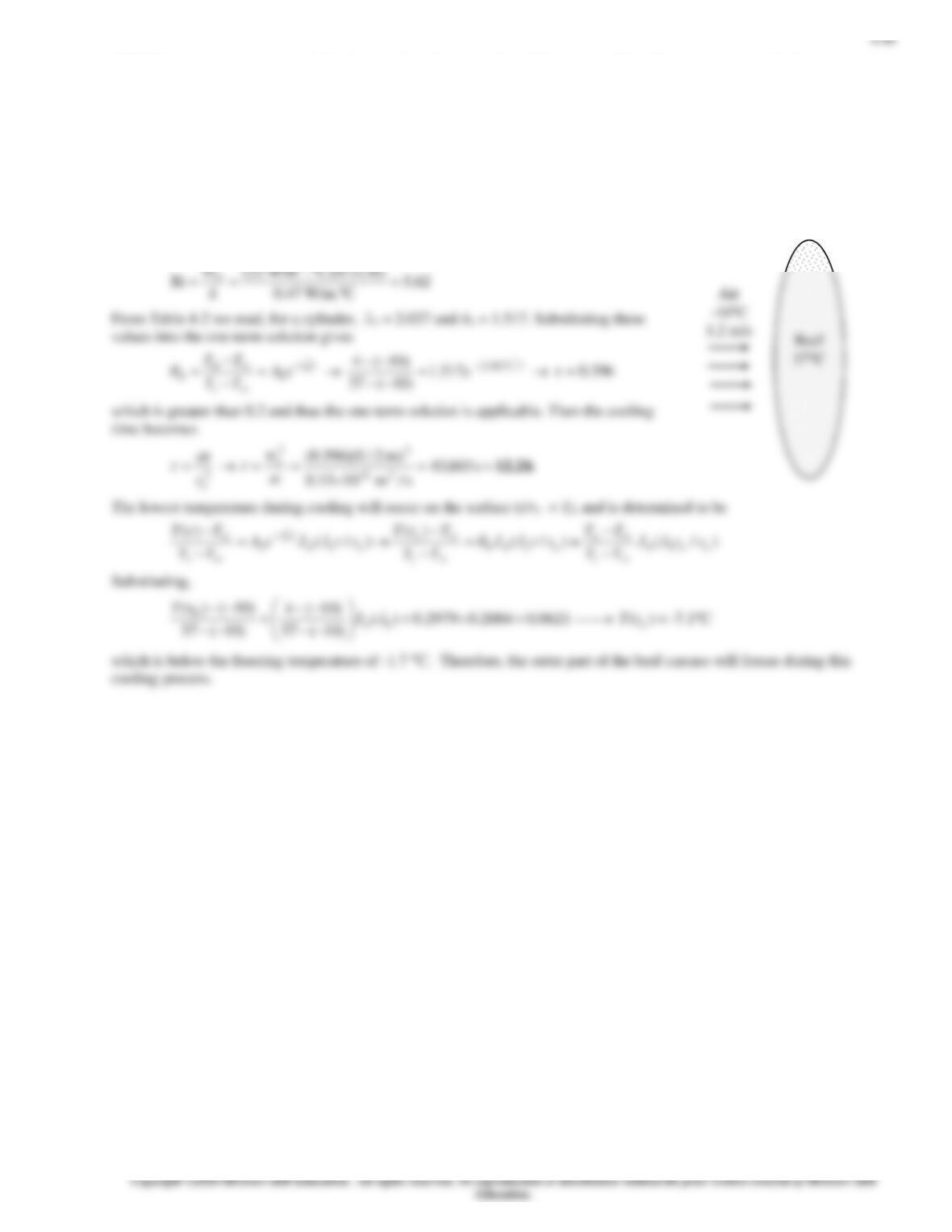4-65 The center temperature of a beef carcass is to be lowered to 4C during cooling. The cooling time and if any part of the
carcass will suffer freezing injury during this cooling process are to be determined.
Assumptions 1 The beef carcass can be approximated as a cylinder with insulated top and base surfaces having a radius of ro
= 12 cm and a height of H = 1.4 m. 2 Heat conduction in the carcass is one-dimensional in the radial direction because of the
symmetry about the centerline. 3 The thermal properties of the carcass are constant. 4 The heat transfer coefficient is constant
and uniform over the entire surface. 5 The Fourier number is > 0.2 so that the one-term approximate solutions are
applicable (this assumption will be verified).
Properties The thermal conductivity and thermal diffusivity of carcass are given to be k = 0.47 W/mC and = 0.1310-6
m2/s.
Analysis First we find the Biot number:
2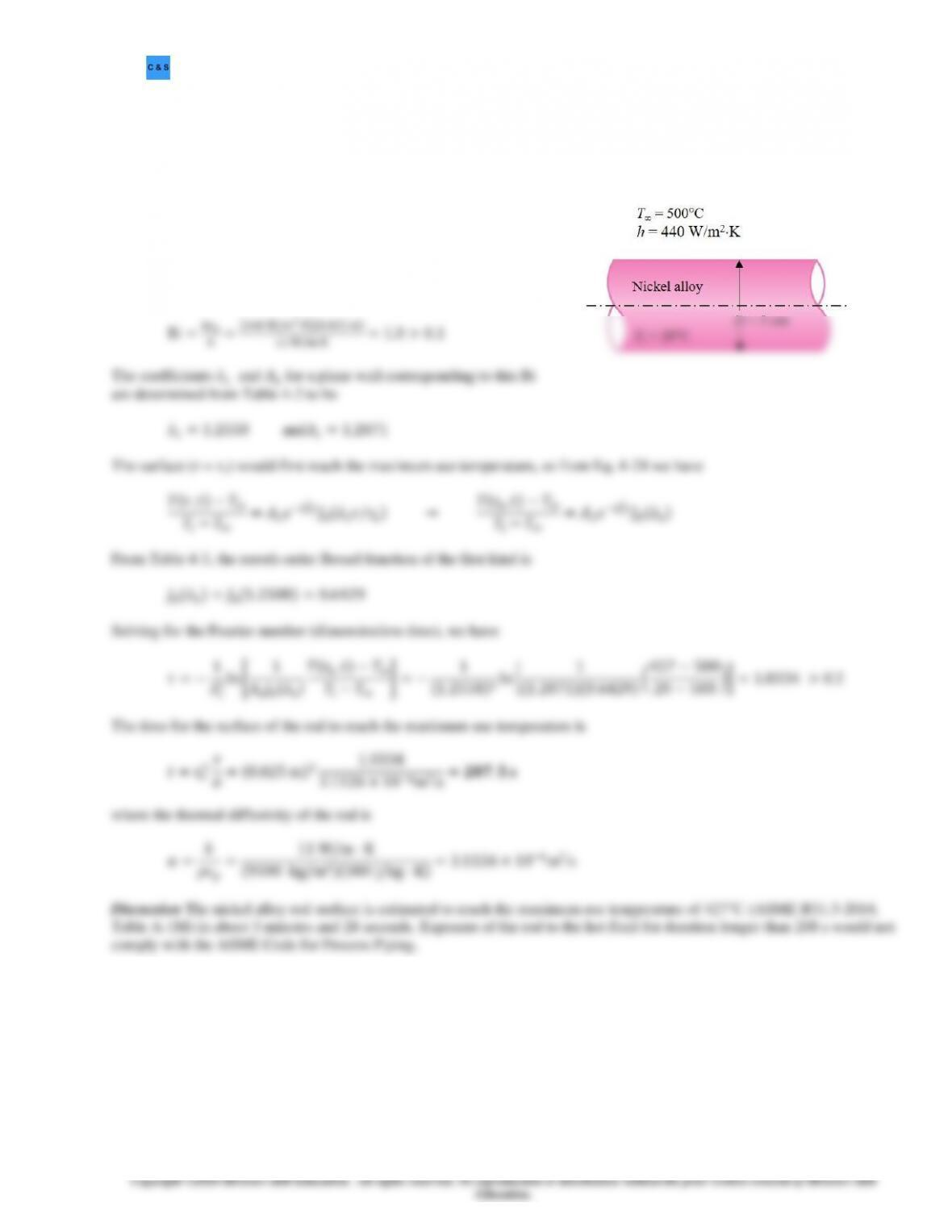4-49
4-66 ASTM B335 rod is submerged in hot fluid at T = 500°C and h = 440 W/m2·K. The rod has an initial temperature
of 20°C. How long can the rod submerge in the hot fluid before reaching its maximum use temperature?
Assumptions1 Heat conduction is transient and one dimensional. 2 Thermal properties are constant. 3 Radiation effects are
negligible. 4 The convection heat transfer coefficient is constant. 5 The Fourier number is > 0.2 so that the one term-term
approximate solutions are applicable.
Properties The thermal properties given are cp = 380 J/kg·K,
k = 11 W/m·K, and ρ = 9300 kg/m3.
Analysis The rod is considered as a long cylinder with a radius of
ro = 25 mm subjected to convection at the surface. The Biot
number for this process is
Bi=ℎ𝑟𝑜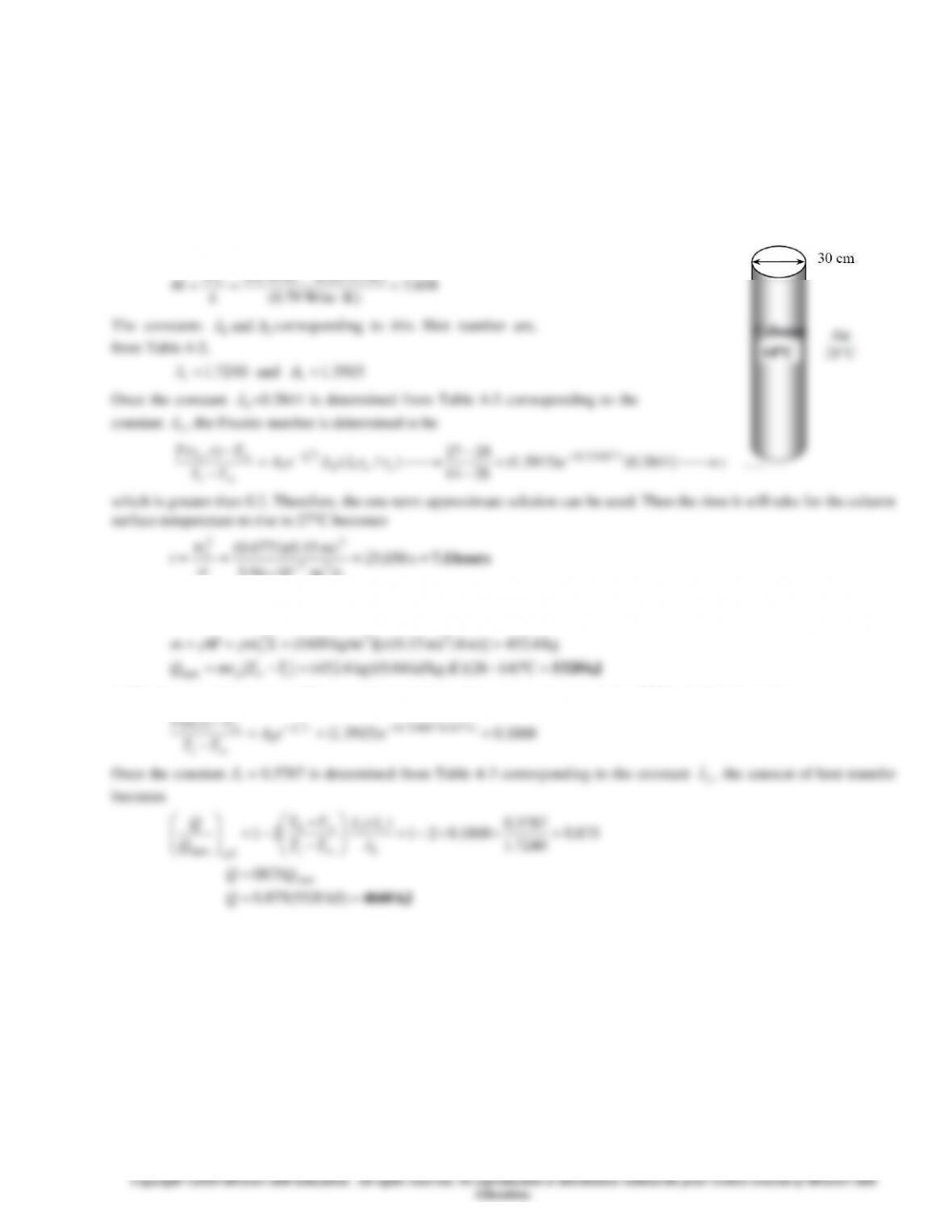4-50
4-67 A cold cylindrical concrete column is exposed to warm ambient air during the day. The time it will take for the surface
temperature to rise to a specified value, the amounts of heat transfer for specified values of center and surface temperatures
are to be determined using the approximate analytical solutions.
Assumptions 1 Heat conduction in the column is one-dimensional since it is long and it has thermal symmetry about the
center line. 2 The thermal properties of the column are constant. 3 The heat transfer coefficient is constant and uniform over
the entire surface. 4 The Fourier number is > 0.2 so that the one-term approximate solutions is applicable (this assumption
will be verified).
Properties The properties of concrete are given to be k = 0.79 W/m.K, = 5.9410-7 m2/s, = 1600 kg/m3 and cp = 0.84
kJ/kg.K
Analysis (a) The Biot number is
)m 15.0)(K W/m14(2
hr
/sm 1094.5
(b) The heat transfer to the column will stop when the center temperature of column reaches to the ambient temperature,
which is 28C. That is, we are asked to determine the maximum heat transfer between the ambient air and the column.
(c) To determine the amount of heat transfer until the surface temperature reaches to 27C, we first determine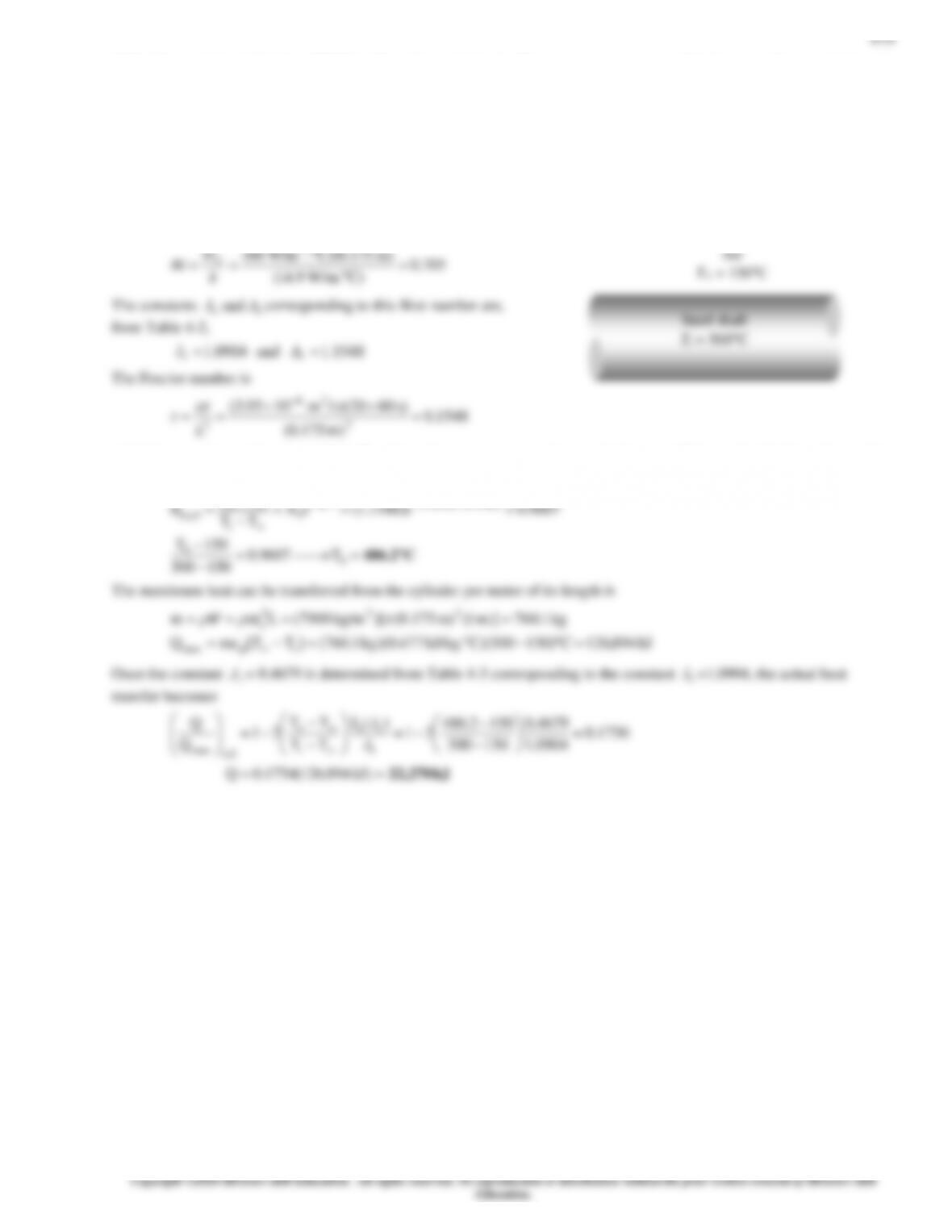4-68 A long cylindrical shaft at 400C is allowed to cool slowly. The center temperature and the heat transfer per unit length
of the cylinder are to be determined.
Assumptions 1 Heat conduction in the shaft is one-dimensional since it is long and it has thermal symmetry about the center
line. 2 The thermal properties of the shaft are constant. 3 The heat transfer coefficient is constant and uniform over the entire
surface. 4 The Fourier number is > 0.2 so that the one-term approximate solutions are applicable (this assumption will be
verified).
Properties The properties of stainless steel 304 at room temperature are given to be k = 14.9 W/m.C, = 7900 kg/m3, cp =
477 J/kg.C, = 3.9510-6 m2/s
Analysis First the Biot number is calculated to be
which is very close to the value of 0.2. Therefore, the one-term approximate solution can still be used, with the understanding
that the error involved will be a little more than 2 percent. Then the temperature at the center of the shaft becomes
)1548.0()0904.1(
0
TT 2
2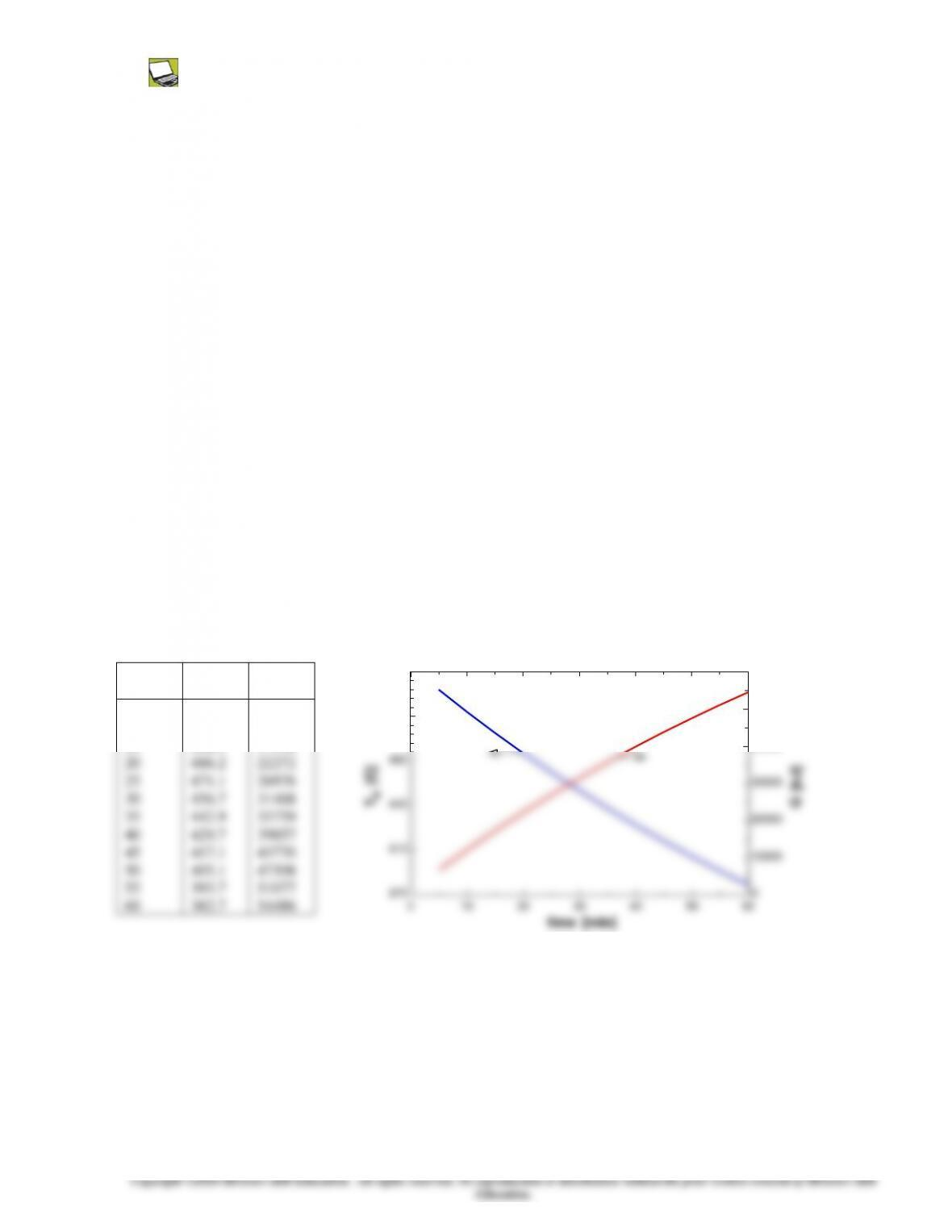4-52
4-69 Prob. 4-68 is reconsidered. The effect of the cooling time on the final center temperature of the shaft and the amount
of heat transfer is to be investigated.
Analysis The problem is solved using EES, and the solution is given below.
"GIVEN"
r_o=(0.35/2) [m]
T_i=500 [C]
T_infinity=150 [C]
h=60 [W/m^2-C]
time=20 [min]
"PROPERTIES"
k=14.9 [W/m-C]
rho=7900 [kg/m^3]
c_p=477 [J/kg-C]
alpha=3.95E-6 [m^2/s]
"ANALYSIS"
Bi=(h*r_o)/k
"From Table 4-2 corresponding to this Bi number, we read"
lambda_1=1.0904
A_1=1.1548
J_1=0.4679 "From Table 4-3, corresponding to lambda_1"
tau=(alpha*time*Convert(min, s))/r_o^2
(T_o-T_infinity)/(T_i-T_infinity)=A_1*exp(-lambda_1^2*tau)
L=1 "[m], 1 m length of the cylinder is considered"
V=pi*r_o^2*L
m=rho*V
Q_max=m*c_p*(T_i-T_infinity)*Convert(J, kJ)
Q/Q_max=1-2*(T_o-T_infinity)/(T_i-T_infinity)*J_1/lambda_1
time
[min]
To
[C]
Q
[kJ]
5
10
15
536
518.7
502.1
6788
12188
17346
515
550
40000
50000
60000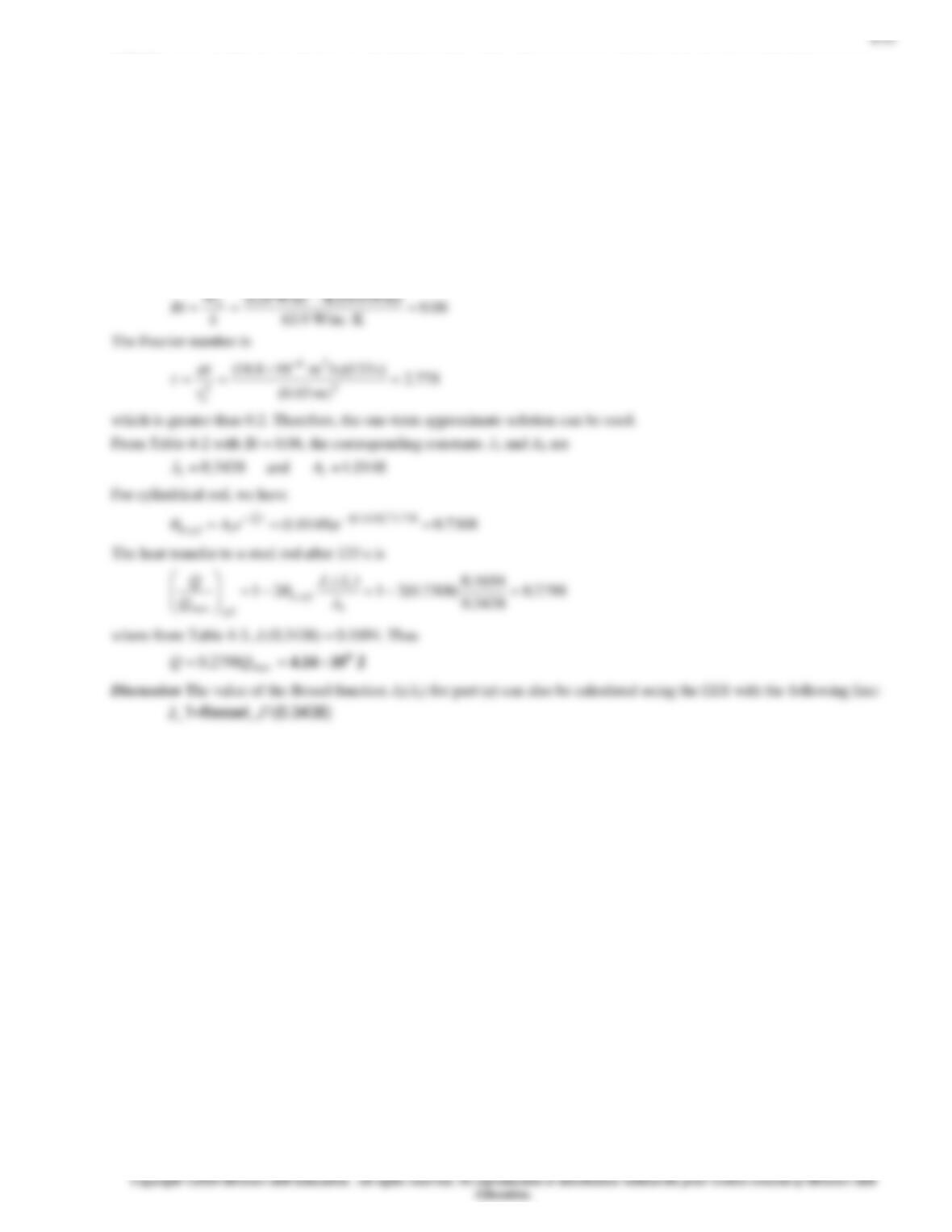4-70 The amount of heat transfer to a steel rod being drawn through an oven is to be determined using analytical one-term
approximate method.
Assumptions 1 Heat conduction is one-dimensional. 2 Thermal properties are constant. 3 Convection heat transfer coefficient
is uniform. 4 Heat transfer by radiation is negligible.
Properties The properties of the steel rod are given as
= 7832 kg/m3, cp = 434 J/kg ∙ K, k = 63.9 W/m ∙ K, and
= 18.8
10−6 m2/s.
Analysis The maximum amount of heat transfer to a steel rod is
J 1048.1K )30800)(KJ/kg 434()m 03.0)(m 2()kg/m 7832(
)()(
723
2
max
==
==

ipoip TTcLrTTcQ V
The Biot number for this process is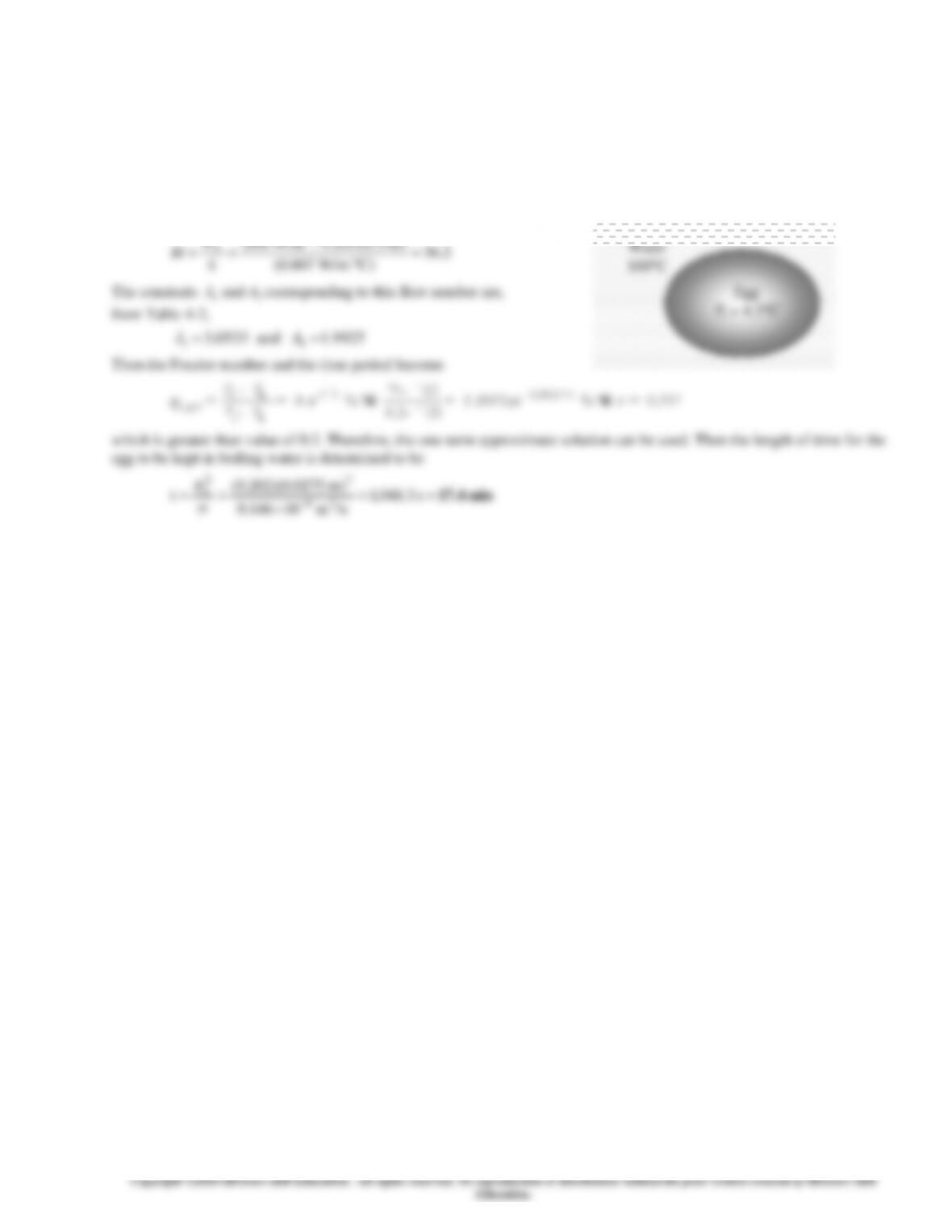4-54
4-71 An egg is dropped into boiling water. The cooking time of the egg is to be determined.
Assumptions 1 The egg is spherical in shape with a radius of r0 = 2.75 cm. 2 Heat conduction in the egg is one-dimensional
because of symmetry about the midpoint. 3 The thermal properties of the egg are constant. 4 The heat transfer coefficient is
constant and uniform over the entire surface. 5 The Fourier number is > 0.2 so that the one-term approximate solutions are
applicable (this assumption will be verified).
Properties The thermal conductivity and diffusivity of the eggs can be approximated by those of water at room temperature
to be k = 0.607 W/m.C,
p
ck
/=
= 0.14610-6 m2/s (Table A-9).
Analysis The Biot number is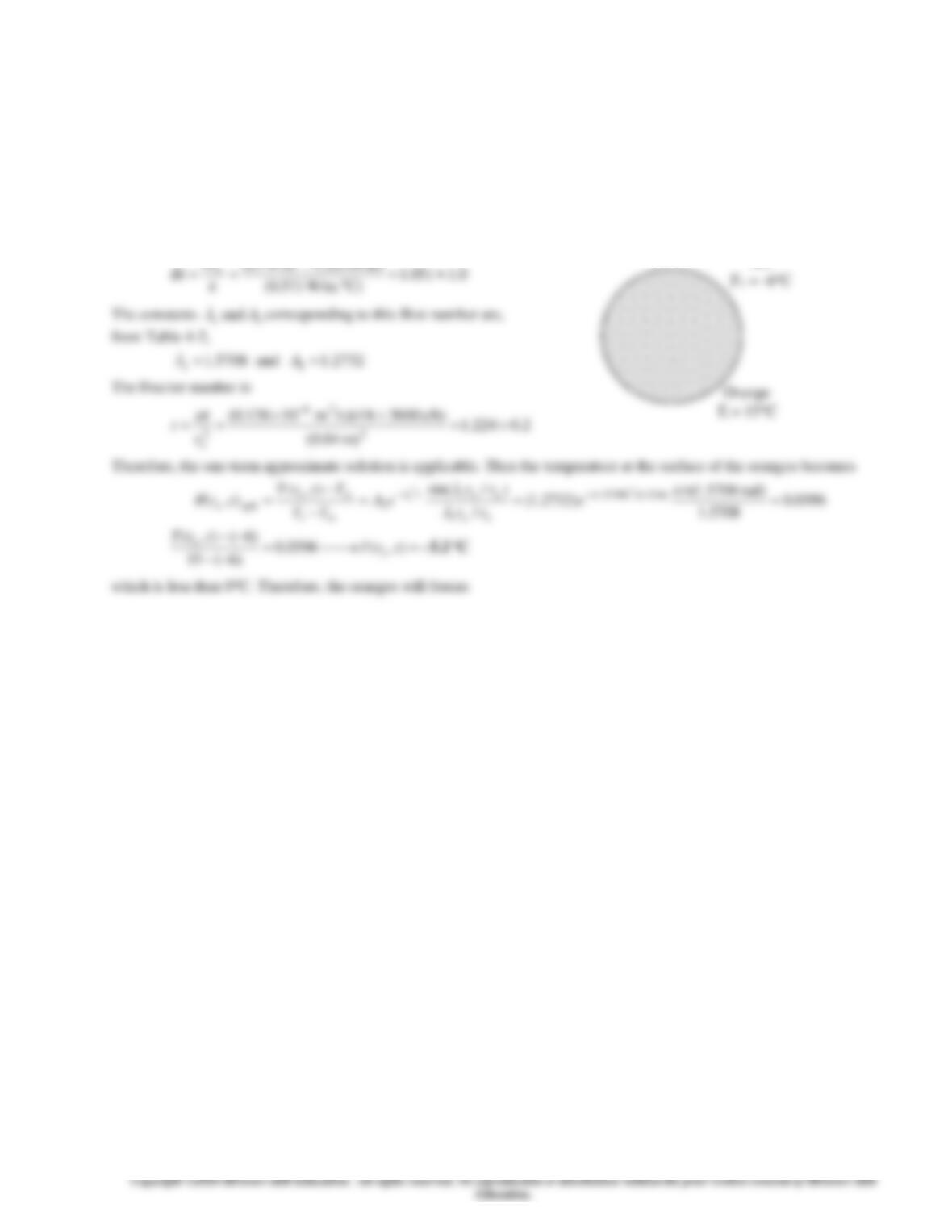4-55
4-72 An orange is exposed to very cold ambient air. It is to be determined whether the orange will freeze in 4 h in subfreezing
temperatures.
Assumptions 1 The orange is spherical in shape with a diameter of 8 cm. 2 Heat conduction in the orange is one-dimensional
because of symmetry about the midpoint. 3 The thermal properties of the orange are constant, and are those of water. 4 The
heat transfer coefficient is constant and uniform over the entire surface. 5 The Fourier number is > 0.2 so that the one-term
approximate solutions are applicable (this assumption will be verified).
Properties The properties of the orange are approximated by those of water at the average temperature of about 5C, k =
0.571 W/m.C and
/sm 10136.0)42059.999/(571.0/ 26
=== p
ck
(Table A-9).
Analysis The Biot number is
hr
Air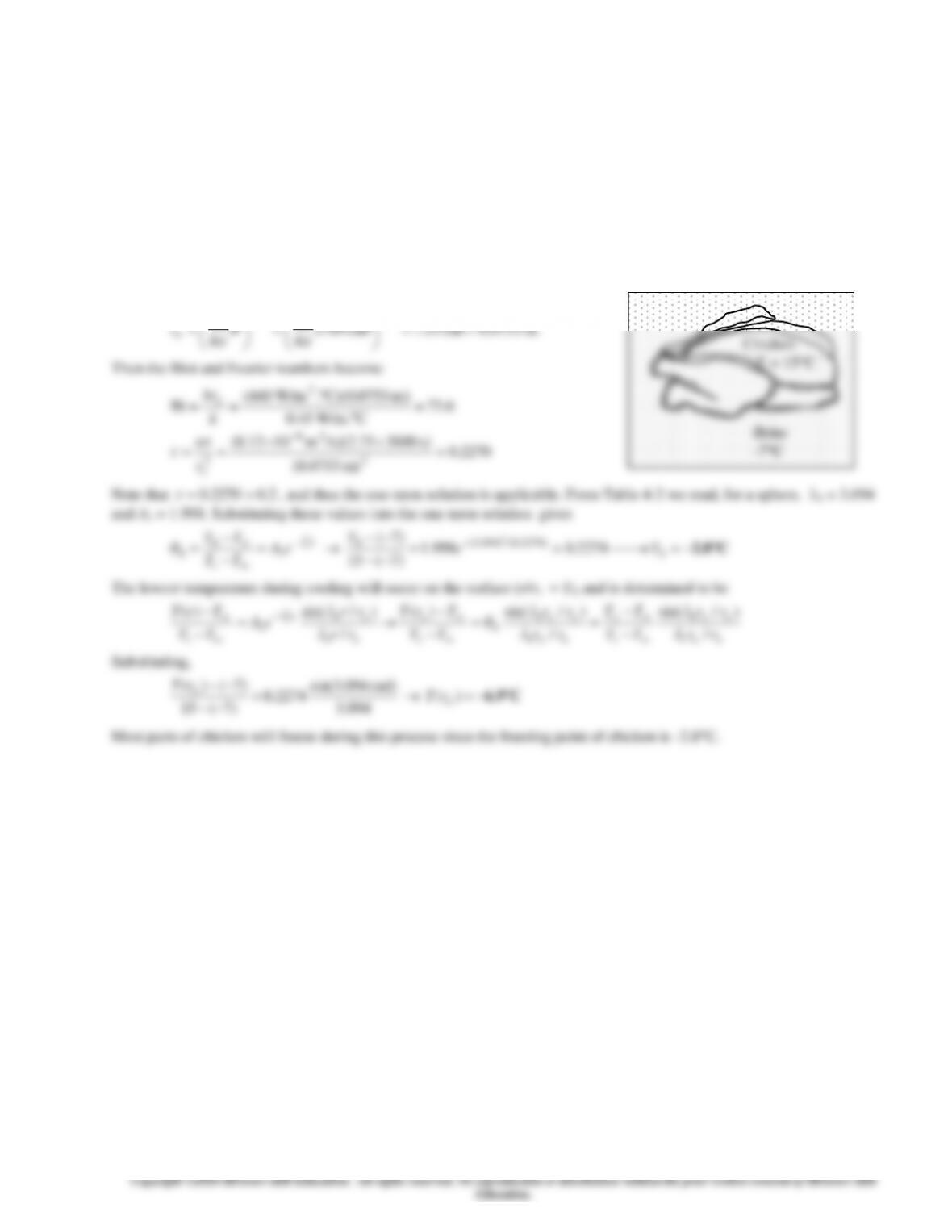4-56
4-73 Chickens are to be chilled by holding them in agitated brine for 2.75 h. The center and surface temperatures of the
chickens are to be determined, and if any part of the chickens will freeze during this cooling process is to be assessed.
Assumptions 1 The chickens are spherical in shape. 2 Heat conduction in the chickens is one-dimensional in the radial
direction because of symmetry about the midpoint. 3 The thermal properties of the chickens are constant. 4 The heat transfer
coefficient is constant and uniform over the entire surface. 5 The Fourier number is > 0.2 so that the one-term approximate
solutions are applicable (this assumption will be verified). 6 The phase change effects are not considered, and thus the actual
the temperatures will be much higher than the values determined since a considerable part of the cooling process will occur
during phase change (freezing of chicken).
Properties The thermal conductivity, thermal diffusivity, and density of chickens are given to be k = 0.45 W/mC, =
0.1310-6 m2/s, and = 950 kg/ m3. These properties will be used for both fresh and frozen chicken.
Analysis We first find the volume and equivalent radius of the chickens:
cm³1789m³)g/(0.95g/c1700/ ===
m
V
3
33/13/1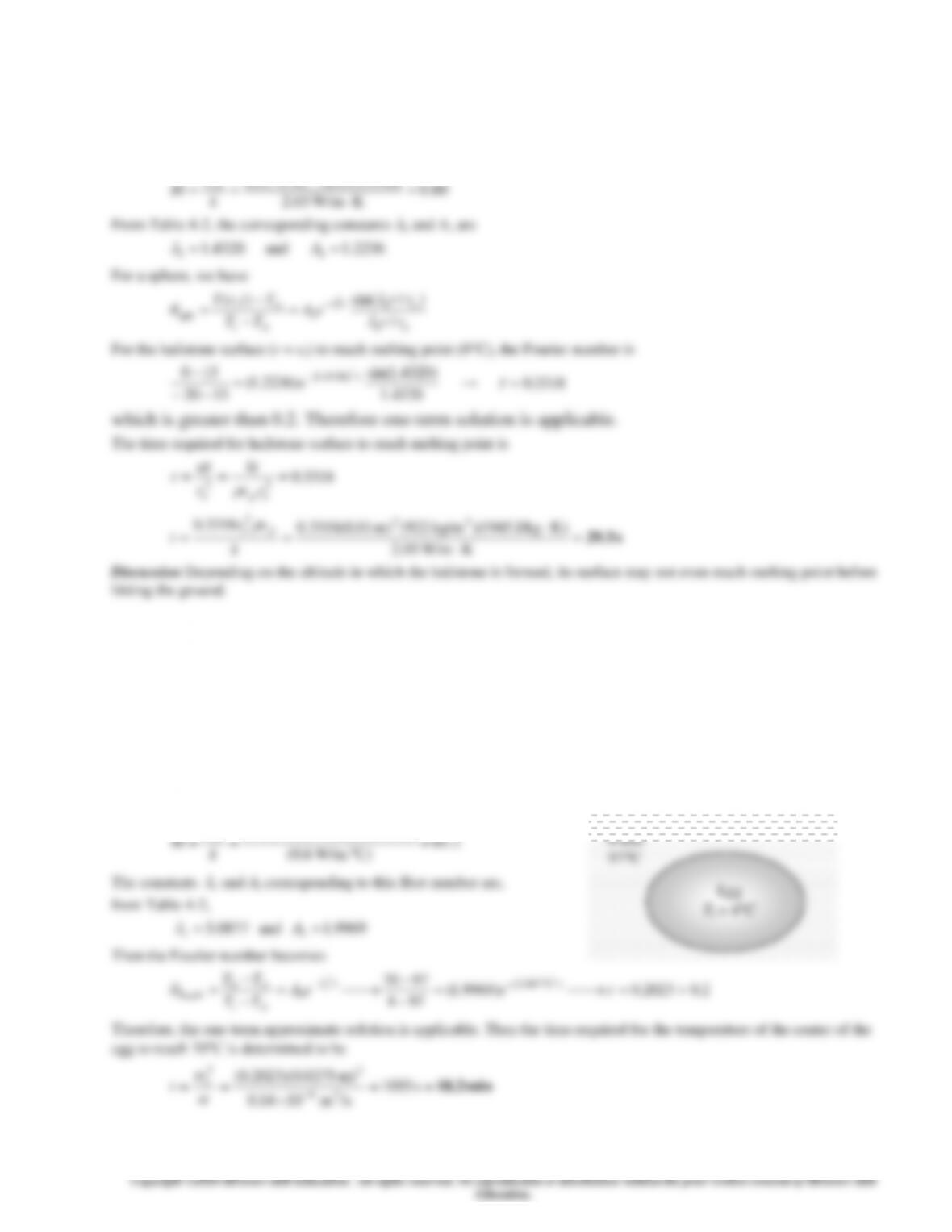4-57
4-74 The time it takes for the surface of a falling hailstone to reach melting point is to be determined.
Assumptions 1 Heat conduction is one-dimensional. 2 Thermal properties are constant. 3 Convection heat transfer coefficient
is uniform. 4 Heat transfer by radiation is negligible.
Properties The properties of ice at 253 K are
= 922 kg/m3, cp = 1945 J/kg ∙ K, and k = 2.03 W/m ∙ K (from Table A-8).
Analysis The Biot number for this process is
)m 010.0)(K W/m163(2
hr
4-75 An egg is dropped into boiling water. The cooking time of the egg is to be determined.
Assumptions 1 The egg is spherical in shape with a radius of ro = 2.75 cm. 2 Heat conduction in the egg is one-dimensional
because of symmetry about the midpoint. 3 The thermal properties of the egg are constant. 4 The heat transfer coefficient is
constant and uniform over the entire surface. 4 The Fourier number is > 0.2 so that the one-term approximate solutions are
applicable (this assumption will be verified).
Properties The thermal conductivity and diffusivity of the eggs are given to be k = 0.6 W/m.C and = 0.1410-6 m2/s.
Analysis The Biot number for this process is
)m 0275.0)(C. W/m1400( 2
hr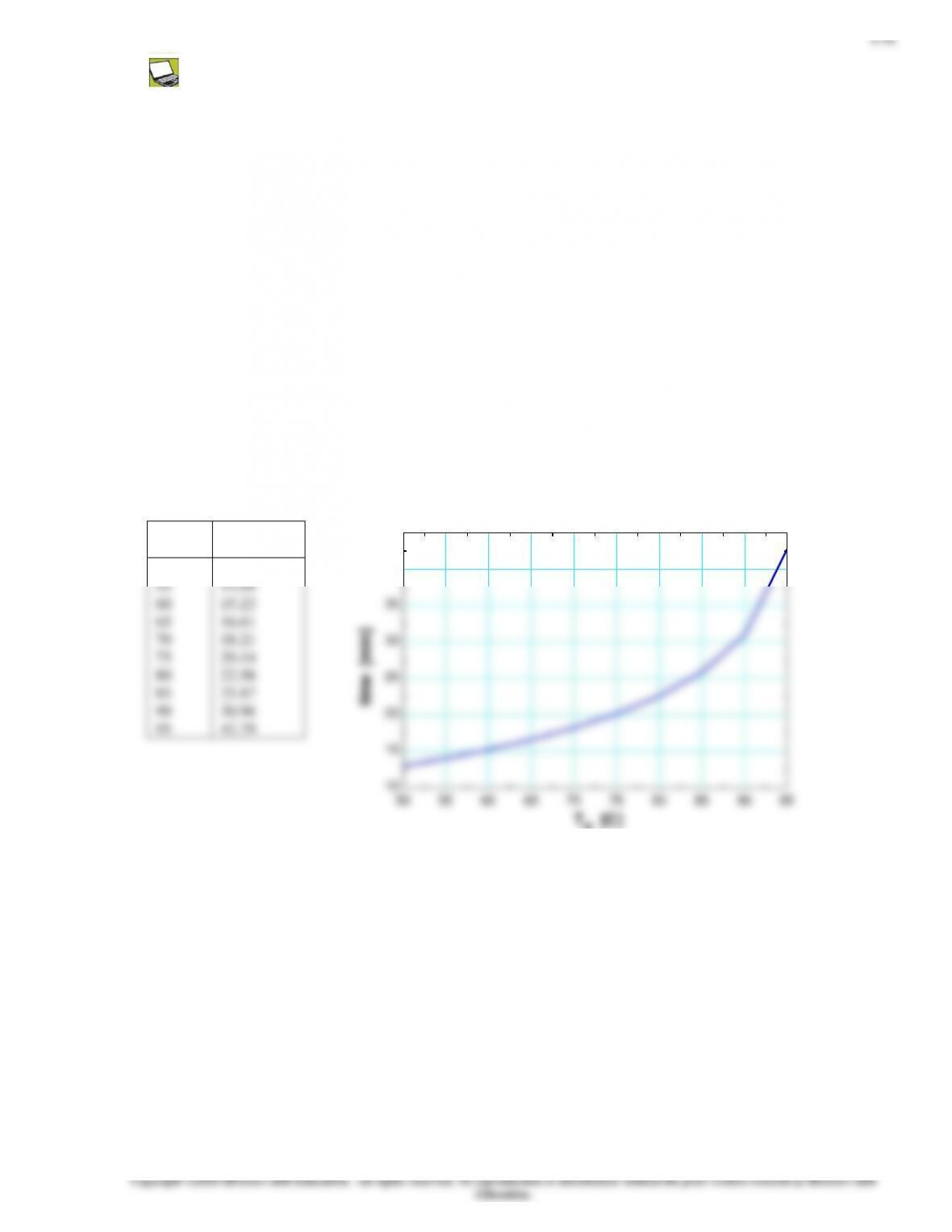4-76 Prob. 4-75 is reconsidered. The effect of the final center temperature of the egg on the time it will take for the
center to reach this temperature is to be investigated.
Analysis The problem is solved using EES, and the solution is given below.
"GIVEN"
D=0.055 [m]
T_i=4 [C]
T_o=70 [C]
T_infinity=97 [C]
h=1400 [W/m^2-C]
"PROPERTIES"
k=0.6 [W/m-C]
alpha=0.14E-6 [m^2/s]
"ANALYSIS"
Bi=(h*r_o)/k
r_o=D/2
"From Table 4-2 corresponding to this Bi number, we read"
lambda_1=1.9969
A_1=3.0863
(T_o-T_infinity)/(T_i-T_infinity)=A_1*exp(-lambda_1^2*tau)
time=(tau*r_o^2)/alpha*Convert(s, min)
To
[C]
time
[min]
50
12.98
40
45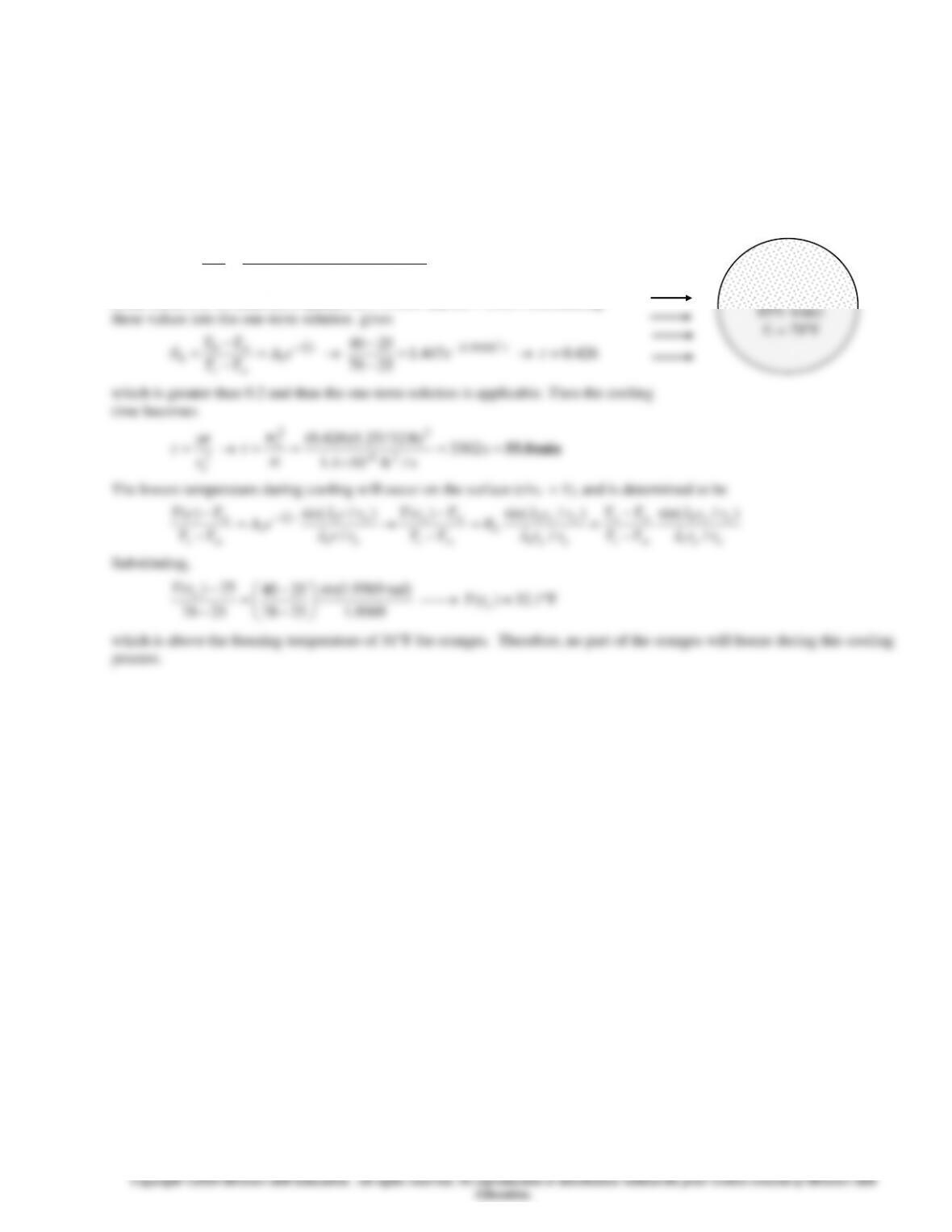4-59
4-77E The center temperature of oranges is to be lowered to 40F during cooling. The cooling time and if any part of the
oranges will freeze during this cooling process are to be determined.
Assumptions 1 The oranges are spherical in shape with a radius of ro =1.25 in = 0.1042 ft. 2 Heat conduction in the orange is
one-dimensional in the radial direction because of the symmetry about the midpoint. 3 The thermal properties of the orange
are constant. 4 The heat transfer coefficient is constant and uniform over the entire surface. 5 The Fourier number is > 0.2
so that the one-term approximate solutions are applicable (this assumption will be verified).
Properties The thermal conductivity and thermal diffusivity of oranges are given to be k = 0.26 Btu/hftF and = 1.410-6
ft2/s.
Analysis First we find the Biot number:
843.1
FBtu/h.ft. 0.26
)ft 12/25.1(F).Btu/h.ft 6.4(
Bi
2
=
== k
hro
From Table 4-2 we read, for a sphere, 1 = 1.9569 and A1 = 1.447. Substituting
Orange
D = 2.5 in
Air
25F
1 ft/s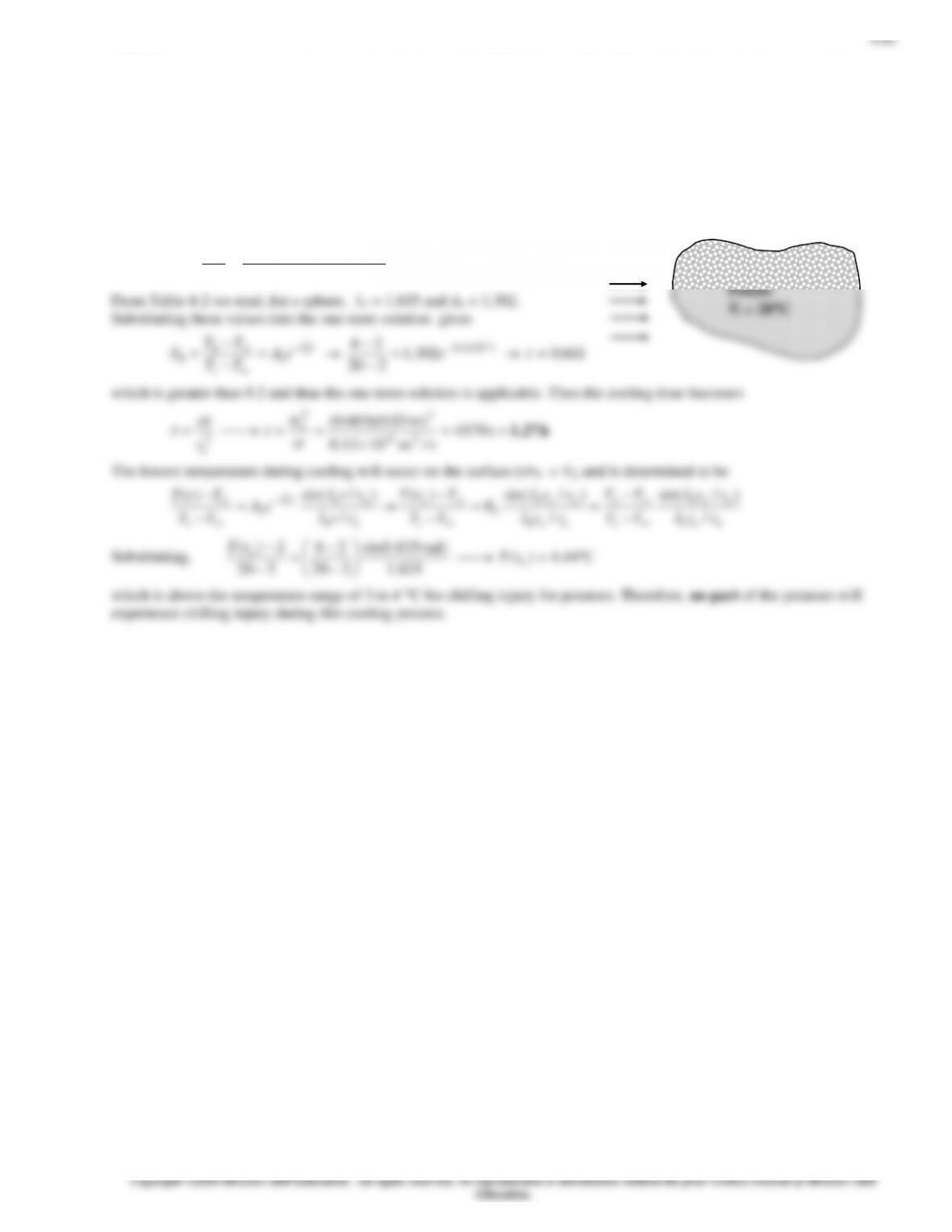4-78 The center temperature of potatoes is to be lowered to 6C during cooling. The cooling time and if any part of the
potatoes will suffer chilling injury during this cooling process are to be determined.
Assumptions 1 The potatoes are spherical in shape with a radius of r0 = 3 cm. 2 Heat conduction in the potato is one-
dimensional in the radial direction because of the symmetry about the midpoint. 3 The thermal properties of the potato are
constant. 4 The heat transfer coefficient is constant and uniform over the entire surface. 5 The Fourier number is > 0.2 so
that the one-term approximate solutions are applicable (this assumption will be verified).
Properties The thermal conductivity and thermal diffusivity of potatoes are given to be k = 0.50 W/mC and = 0.1310-6
m2/s.
Analysis First we find the Biot number:
14.1
C. W/m0.5
)m 03.0(C). W/m19(
Bi
2
=
== k
hro
Air
2C
4 m/s